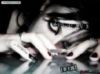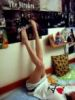ofps4c25 : ­Ó¤HĄD­¶ - şô¤W¤é»x - ¬ŰĂŻ ¤Ŕ¨É¦ÜFACEBOOK   ------ ¦n¤Í¬ŰĂŻ ------ ¶W¶W=] Şş¬ŰĂŻ şµżß­ô­ô=] Şş¬ŰĂŻ ¤X®Ę=] Şş¬ŰĂŻ Aroma=] Şş¬ŰĂŻ aR_YeE_baby Şş¬ŰĂŻ ¤XŔÚ=] Şş¬ŰĂŻ ¬n¬n=] Şş¬ŰĂŻ Baby KoMi=] Şş¬ŰĂŻ ÄRÄR=] Şş¬ŰĂŻ BoBo=] Şş¬ŰĂŻ ¤T¤ĺł˝=] Şş¬ŰĂŻ żßżß=] Şş¬ŰĂŻ «ä=] Şş¬ŰĂŻ ±Ó±Ó=] Şş¬ŰĂŻ ¤X«Ľ=] Şş¬ŰĂŻ ¤X¸Ö=] Şş¬ŰĂŻ Mandy=] Şş¬ŰĂŻ µŻ¦Ě=] Şş¬ŰĂŻ ·©·© Şş¬ŰĂŻ coybaby_63 Şş¬ŰĂŻ CrazyKO Şş¬ŰĂŻ ¤BBB=] Şş¬ŰĂŻ CYT_Doris Şş¬ŰĂŻ kenny=] Şş¬ŰĂŻ KinGĄJ=] Şş¬ŰĂŻ Emily©j=] Şş¬ŰĂŻ ŔÜ=] Şş¬ŰĂŻ 5+2=] Şş¬ŰĂŻ ­SĄJ=] Şş¬ŰĂŻ ¤Xľĺ=] Şş¬ŰĂŻ fisHba1L Şş¬ŰĂŻ fishfish0522 Şş¬ŰĂŻ ŞYŞY=] Şş¬ŰĂŻ ¤XŞĺ=] Şş¬ŰĂŻ ®a©j=] Şş¬ŰĂŻ ¤p§Í=] Şş¬ŰĂŻ janicekb Şş¬ŰĂŻ 7°A=] Şş¬ŰĂŻ żĽżĽ=] Şş¬ŰĂŻ ¤XŔÜ=] Şş¬ŰĂŻ joseph_wonG Şş¬ŰĂŻ ®aĄß=] Şş¬ŰĂŻ ĄĚ¤jĄi=] Şş¬ŰĂŻ Żä®¦=] Şş¬ŰĂŻ ¤XĽÖ=] Şş¬ŰĂŻ kenwong987 Şş¬ŰĂŻ kiki=] Şş¬ŰĂŻ killuawu_ Şş¬ŰĂŻ ¤X¶Ż=] Şş¬ŰĂŻ ŔR=] Şş¬ŰĂŻ Ěô=] Şş¬ŰĂŻ ¤X°a=] Şş¬ŰĂŻ ¤X®Ô=] Şş¬ŰĂŻ ¤p©ż=] Şş¬ŰĂŻ bee fd Şş¬ŰĂŻ Jacqueline=] Şş¬ŰĂŻ ŔÜ¤l­ô=] Şş¬ŰĂŻ ÂfÂc=] Şş¬ŰĂŻ leung816 Şş¬ŰĂŻ June=] Şş¬ŰĂŻ §ü¶Ě=] Şş¬ŰĂŻ »}=] Şş¬ŰĂŻ louis=] Şş¬ŰĂŻ ˛˘˛˘°é=] Şş¬ŰĂŻ ¤ő®ăB=] Şş¬ŰĂŻ ¤XBee=] Şş¬ŰĂŻ ŞY=] Şş¬ŰĂŻ żcżc=] Şş¬ŰĂŻ ¤XĄ­=] Şş¬ŰĂŻ °A=] Şş¬ŰĂŻ ż}=] Şş¬ŰĂŻ ł·ż|=] Şş¬ŰĂŻ Man_0108 Şş¬ŰĂŻ May=] Şş¬ŰĂŻ şűĄLĄ¤=] Şş¬ŰĂŻ Milk_yung Şş¬ŰĂŻ MingTin Şş¬ŰĂŻ ¤H§Ż=] Şş¬ŰĂŻ nitky2333 Şş¬ŰĂŻ peterzacs Şş¬ŰĂŻ pomelo_pui Şş¬ŰĂŻ 宝宝=] Şş¬ŰĂŻ ŻE=] Şş¬ŰĂŻ Rainbow=] Şş¬ŰĂŻ ĄiĄi=] Şş¬ŰĂŻ rainine1996 Şş¬ŰĂŻ ca±ů=] Şş¬ŰĂŻ sammi_l0ve Şş¬ŰĂŻ ¤×ł˝=] Şş¬ŰĂŻ seed777 Şş¬ŰĂŻ ¬Ŕ宝宝=] Şş¬ŰĂŻ sheila_1028 Şş¬ŰĂŻ ¤X©f=] Şş¬ŰĂŻ Ŕs=] Şş¬ŰĂŻ §Í=] Şş¬ŰĂŻ ¤XŔR=] Şş¬ŰĂŻ superwoman95 Şş¬ŰĂŻ ŻřŔY=] Şş¬ŰĂŻ ´µ´µ Şş¬ŰĂŻ ¤XwInG=] Şş¬ŰĂŻ ¬Áłí=] Şş¬ŰĂŻ ´Ł¤l=] Şş¬ŰĂŻ ¤XąF=] Şş¬ŰĂŻ Tong=] Şş¬ŰĂŻ Tracy=] Şş¬ŰĂŻ ąĘ¤j·Ý=] Şş¬ŰĂŻ ąa=] Şş¬ŰĂŻ TszYing5201 Şş¬ŰĂŻ ¤ĺ=] Şş¬ŰĂŻ ĄdµáŞM=] Şş¬ŰĂŻ wanwankobe Şş¬ŰĂŻ wat3rbabii Şş¬ŰĂŻ wongchingki Şş¬ŰĂŻ ˛¦˛¦=] Şş¬ŰĂŻ ĄCĄC=] Şş¬ŰĂŻ YeeJoey Şş¬ŰĂŻ ¶ş¶ş=] Şş¬ŰĂŻ Ľä¨ŕ=] Şş¬ŰĂŻ YuezB Şş¬ŰĂŻ ¤pŻů=] Şş¬ŰĂŻ ¤X©y=] Şş¬ŰĂŻ Mr.»ć=] Şş¬ŰĂŻ ¤p¶Ż=] Şş¬ŰĂŻ ofps4c25Şş¬ŰĂŻ > «DĄD¬y > 5 ąĎ¤ů¸ę°T | ŔËµř­ěąĎ | µoŞíŻd¨Ą | ¦^¤W¤@Ľh53 4 5 6 7